# Methods and formulas for CUSUM Chart

Select the method or formula of your choice.

## CUSUM plan, h, k, and FIR

With in-control processes, CUSUM charts are good at detecting small shifts away from the target, because they incorporate information from the sequence of sample values. The plotted points are the cumulative sums of the deviations of the sample values from the target. These points should fluctuate randomly around zero. If a trend develops upwards or downwards, it should be considered as evidence that the process mean has shifted, and you should look for special causes.

Minitab generates two kinds of CUSUMs.
Tabular CUSUM (the default)
The upper CUSUM detects upward shifts in the level of the process and the lower CUSUM detects downward shifts. This chart uses control limits (UCL and LCL) to determine when an out-of-control situation has occurred. See Prins et al.1 and Stoumbos et al.2 for a discussion of tabular CUSUMs.
This chart uses a V-mask, rather than control limits, to determine when an out-of-control situation has occurred. See Lucas3 and Wadsworth et al.4 for a discussion of the V-mask chart.

CUSUM charts are defined by two parameters, h and k, which are often referred to as the CUSUM plan. These values are often selected from ARL (Average Run Length) tables. See Lucas3 and Lucas et al.5.

### h

For tabular CUSUMs, h is the number of standard deviations between the center line and the control limits. It is the value at which an out-of-control signal occurs.

For V-mask CUSUMs, Minitab calculates the half-width of the V-mask (H) at the point of origination by H = .

The default value for h is 4.

### k

For tabular CUSUMs, k is the allowable "slack" in the process. In the CUSUM point formula, k specifies the size of the shift you want to detect.

For V-mask CUSUMs, k is the slope of the V-mask arms. You can select k using an ARL table.

The default value for k is 0.5.

### FIR

FIR (fast initial response) is a method used to initialize the tabular CUSUM. Normally, tabular CUSUMs are initialized at 0, but if the process is out of control at startup, the CUSUMs will not detect the situation for several subgroups.

## Tabular CUSUM

### Plotted points

The data plotted in a CUSUM chart are CLi, CUi.

The value of a lower tabular CUSUM at time i:

where:

The value of an upper tabular CUSUM at time i:

where:

### Center line

For the default, tabular CUSUM chart, the center line is 0.

### Notation

TermDescription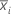subgroup mean
T target
ksize of the shift you want to detect
σ process standard deviation
m subgroup size
f FIR
h decision interval

### Plotted points

Ci, the value of a V-mask CUSUM at time i =

where C0 = 0

The default chart uses n to estimate the origin p.

### Notation

TermDescription
T target
h decision interval
msubgroup size

## Methods and formulas for Box-Cox

### Box-Cox formula

If you use a Box-Cox transformation, Minitab transforms the original data values (Yi) according to the following formula:

where λ is the parameter for the transformation. Minitab then creates a control chart of the transformed data values (Wi). To learn how Minitab chooses the optimal value for λ, go to Methods and formulas for Box-Cox Transformation.

### Common λ values

The following table shows some commonly used λ values and their transformations.
λ Transformation
2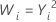0.5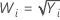0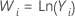−0.5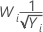−1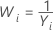1 J. Prins and D. Mader (1997−98). "Multivariate Control Charts for Grouped and Individual Observations", Quality Engineering, 10, 49- 57.
2 Z. Stoumbos, M.R. Reynolds, T.P. Ryan, and W.H. Woodall (2000). "The State of Statistical Process Control as We Proceed into the 21st Century", Journal of the American Statistical Association, 95, 992−998.
3 J.M. Lucas (1976). "The Design and Use of V-Mask Control Schemes", Journal of Quality Technology, 8, 1−12.
4 H.M. Wadsworth, K.S. Stephens, and A.B. Godfrey (2001). Modern Methods for Quality Control and Improvement, 2nd edition, John Wiley & Sons.
5 J.M. Lucas and R.B. Crosier (1982). "Fast Initial Response for CUSUM Quality-Control Schemes: Give Your CUSUM a Head Start", Technometrics, 24, 199−205.
By using this site you agree to the use of cookies for analytics and personalized content.  Read our policy